# Three Dimensional (3D)Shapes | Meaning and Properties of Three Dimensional (3D) Shapes | Different between 2D and 3D Shapes Primary 4 (Basic 4) Term 3 Week 10 Mathematics

### MATHEMATICS

THIRD TERM

WEEK 10

PRIMARY 4

THEME –  GEOMETRY

PREVIOUS LESSON – Lines of Symmetry on Plane Shapes | Vertical and Horizontal Lines | Cardinal Points | Types of Angles Primary 4 (Basic 4) Term 3 Week 9 Mathematics

#### LEARNING AREA

1. Introduction

2. Meaning and Properties of 3D Shapes

3. Different between 2D and 3D Shapes

4. Lesson Evaluation and Weekly Assessment

#### LEARNING OBJECTIVES

By the end of the lesson, most pupils should have attained the following objectives –

1. explain the meaning of 3 dimensional shapes.

2. differentiate between 2D and 3D shapes.

3. identify and state the properties of 3 dimensional shapes.

4. state the uses of three dimensional shapes.

5. apply 3 dimensional shapes in real life situations.

#### ENTRY BEHAVIOUR

The pupils can identify and describe shapes such as tin, cube of sugar and box (commonly known as carton)

#### INSTRUCTIONAL MATERIALS

The teacher will teach the lesson with the aid of sample and chart showing 3 dimensional shapes.

#### METHOD OF TEACHING

Choose a suitable and appropriate methods for the lessons.

Note – Irrespective of choosing methods of teaching, always introduce an activities that will arouse pupil’s interest or lead them to the lessons.

#### REFERENCE MATERIALS

1. Scheme of Work

2. 9 – Years Basic Education Curriculum

3. Course Book

4. All Relevant Material

5. Online Information

### CONTENT OF THE LESSON

#### LESSON 1 – INTRODUCTION

ACTIVITY 1 – 2D AND 3D SHAPES

Three dimensional shapes are objects or shapes that three dimensions such as length, width, and height.

Three dimensional shapes are known as 3D shapes.

While two dimensional shapes have just two dimensions such as length and width.

Two dimensional shapes are also known as 2D shapes.

The properties of a three dimensional shapes are,

1. Faces

2. Edges

3. Vertices

ACTIVITY 2 – THREE DIMENSIONAL SHAPES

The following shapes are the basic three dimensional shapes we see around us,

1. Cube

2. Cuboid (Rectangular prism)

3. Triangular prism

4. Sphere

5. Cone

6. Cylinder

7. Pyramid

#### LESSON 2 – CUBE AND CUBOID

1. CUBE

A cube is a solid three dimensional shape which has 6 square faces.Examples of cube are ice cube, cube square, dice, etc.

The cube has the following properties,

• 8 vertices (corners)
• 12 edges
• 6 faces

2. CUBOID

A cuboid is a solid three dimensional shape which has 6 rectangular faces or 4 rectangular faces and 2 square faces.Six rectangular facesFour rectangular faces with two square faces

Examples of cuboid are the lunch box, bricks, books, carton, cupboard, fridge, etc.

It is also called a rectangular prism.

The faces of the cuboid are a rectangle in shape.

The cuboid has the following properties,

• 8 vertices (corners)
• 12 edges
• 6 f aces

#### LESSON 3 – TRIANGULAR PRISM, SPHERE AND CONE

3. TRIANGULAR PRISM

A triangular prism is a solid three dimensional shape which has 5 faces (2 triangles and 3 rectangles).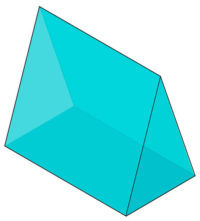Examples of triangular prism are triangular roofs, camping tents, and chocolate candy bars.

The triangular prism has the following properties,

• 6 vertices
• 9 edges
• 5 faces

4. SPHERE

A sphere is a three dimensional round shaped object with just one face.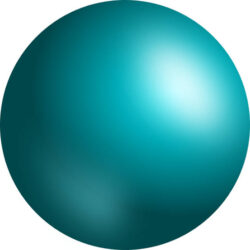Examples of sphere ball, moon, sun, eyeball, orange, etc.

The sphere has the following properties,

• No vertex
• No edges
• 1 curved face

5. CONE

A cone is a three dimensional object or solid with a circular base and has a single vertex.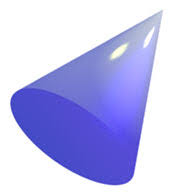Examples of cone are hat, Ice cream cone, funnel, megaphone, christmas tree, traffic cone, etc.

The cone has the following properties,

• 1 vertex
• 1 edge
• 1 flat face – circle
• 1 curved face

#### LESSON 4 – CYLINDER AND PYRAMID

6. CYLINDER

A cylinder is defined as a three dimensional shapes with 2 circular bases connected by a curved surface.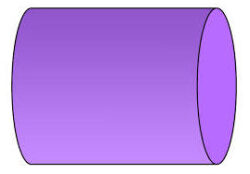Examples of cylinder pipes, cans, tanks, battery, gas cylinder, fire extinguisher, etc.

The cylinder has the following properties,

• No vertex
• 2 edges
• 2 flat faces – circles
• 1 curved face

7. PYRAMID

A pyramid is also a three dimensional shape with a square base and four triangular faces.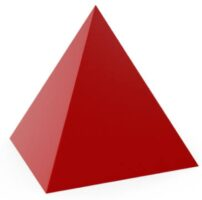Examples of pyramid are roof of a building, Egyptian pyramid, groundnut pyramid, etc.

There are different types of pyramid depending on their based.

We have the triangular, square, quadrilateral base but the most commonly used is the square pyramid.

The pyramid has the following properties,

• 5 vertices
• 8 edges
• 5 faces

LESSON 5 – REVISION AND WEEKLY ASSESSMENT

As stated in performance objectives or lesson evaluation.

#### PRESENTATION

To deliver the lesson, the teacher adopts the following steps:

1. To introduce the lesson, the teacher revises the previous lesson. Based on this, he/she asks the pupils some questions;

2. Teacher organizes the pupils in groups or pair depending on the size of the class.

3. Teacher displays sample and chart of 2D and 3D shapes.

4. Teacher asks pupils as groups or pairs to identify and describe the shapes.

Pupil’s Activities – identify and describe the 2D and 3D shapes.

5. Teacher uses the sample, chart and pupil’s responses to introduce the lesson and leads a discussion on the meaning and different between 2D and 3D shapes with their properties.

Pupil’s Activities – Identify the basic features of 2D and 3D shapes.

6. Teacher uses sample, chart and some of the available real objects of 3 dimensional shapes to discuss the different types of three dimensional shapes.

Pupil’s Activities – Identify and describe three dimensional shapes in their communities.

7. Teacher summarizes the lesson on the board with appropriate evaluation.

Pupil’s Activities – Participate actively in the summary of the lesson and write as instructed.

#### CONCLUSION

To conclude the lesson for the week, the teacher revises the entire lesson and links it to the following week’s lesson.

#### LESSON EVALUATION

1. explain the meaning of 3 dimensional shapes.

2. differentiate between 2D and 3D shapes.

3. identify and state the properties of 3 dimensional shapes.

4. state the uses of three dimensional shapes.

5. apply 3 dimensional shapes in real life situations.

6. state the properties of the following shapes,

• Cube
• Cuboid
• Triangular prism
• Cone
• Sphere
• Pyramid
• Cylinder